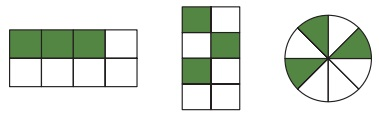Home | | Maths 4th Std | Able to Define fractions

# Able to Define fractions

A fraction is how many part or parts of a whole we have. Fraction = Numerator / Denominator = Collected portion / Divided portion

Able to Define fractions:

Swathi is studying fourth standard, today is her birthday. so the whole class bought a birthday cake for her. In front of her class teacher and students, swathi Cuts her birthday cake. In her class 19 students and a teacher. So she cuts the cake.

Teacher: students, you bought a cake, here cake is a whole part. Swathi divided the whole into 20 equal parts, everybody had a part (piece).

A piece of cake given to one student is one out of twenty. We can write it as 1/20

If we have number’s 1/20 like this, then we Call it as fraction.

In 1/20, 1 is numerator, 20 is denominator.

Thus Combination of number like numerator and denominator is called as fraction.

A fraction is how many part or parts of a whole we have.

Fraction = Numerator / Denominator = Collected portion / Divided portionObserve the following pictures:A circle is divided into four equal parts. In this four parts only one part is shaded.

The shaded portion is fraction = 1/4 (or) one fourth

Definition:

Division of the whole:The whole portion was divided into two equal parts. In this two parts one part is coloured or shaded. The shaded parts fraction = ½ (half)The whole portion was divided into three equal parts. In that two parts are shaded. The shaded parts fraction = 2/3 (two third).The whole portion was divided into four equal parts. In that two parts are shaded. The shaded parts fraction = 2/4 (two fourth)The whole portion was divided into eight equal parts. In that three parts are shaded. The shaded portion’s fraction = 3/8 (Three eighth) 1/2, 2/3, 2/4, 3/8 Such numbers are examples for fraction.

Tags : Fraction | Term 2 Chapter 6 | 4th Maths , 4th Maths : Term 2 Unit 6 : Fraction
Study Material, Lecturing Notes, Assignment, Reference, Wiki description explanation, brief detail
4th Maths : Term 2 Unit 6 : Fraction : Able to Define fractions | Fraction | Term 2 Chapter 6 | 4th Maths